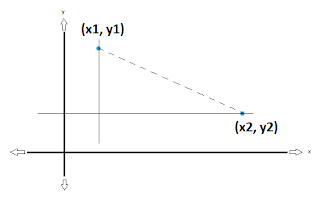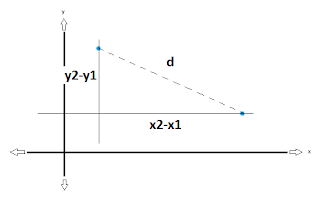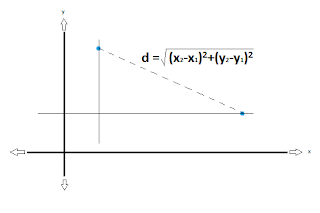# The Distance Formula

This post is going to explain the distance formula: what it is, and where it comes from. When you see how to derive it, you won't need to worry about memorizing the formula anymore. And it's much easier than you think, despite looking kind of scary.  Please click the +1 button if this post helps you!

The distance formula is used to find the exact distance between two points, and can be easily explained with a right angle triangle to demonstrate it.

Assume that you want to find the distance between two points.  If you extend a horizontal line across from one point, and a vertical line through the other, these two lines will intersect at a right angle. You can then imagine the hypotenuse of this right angle triangle to be the distance between the two points in question.From this, you can see that the distance between the two points is simply the length of the hypotenuse.

We already know that to find the length of the hypotenuse, we apply the Theorem of Pythagoras, which says that c2 = a2 + b2. (The square of the hypotenuse is equal to the sum of the squares of the remaining two sides). So from this, then, we see that we need to determine the lengths of the two sides that we have created by extending lines through our points to join at a right angle. And to find these lengths, all we need to know are the coordinates of our two points!

For a general triangle, then, we have something like this:To find the horizontal length, it is just the difference between the two x-coordinates (ie. x2-x1). Think of it as taking a stick that is x2 units long, and chopping off a length of that stick that is x1 units long. The stick that you are left with, x2-x1, is the length of our horizontal side.

The same reasoning applies to find the vertical length, which is the difference between the two y-coordinates (ie. y2-y1). One comment I will make here, is that since we are talking about a length of a side, the length has to be the absolute difference between the two points (ie. you can't have a negative side length).

So then, for our general triangle, we have our two lengths, and let's call the hypotenuse "d" (as in, the distance between the two points).So then, if we apply the Theorem of Pythagoras to this triangle we have created, we can come up with the distance formula very easily!

c2 = a2 + b2...... which we can change to read:
d2 = (x2-x1)2 + (y2-y1)2

And so we have:

d = sqrt [(x2-x1)2 + (y2-y1)2]Let's quickly try with 2 points. You can draw the triangle out as I have above to follow along more closely. I will just do the quick calculation for you though.

Find the distance between the points (1,2) and (3,5).

d = sqrt [(3-1)2 + (5-2)2]
d = sqrt [(2)2 + (3)2]
d = sqrt [4 + 9]
d = sqrt 

And that's all there is to it. I hope that with this example calculation, I've been able to clearly explain how to derive the distance formula.  Once you know where a lot of these formulas come from, you'll never have to worry about memorizing them again!  If you learn how the derivation works first, then in time, you will automatically remember the formula.